Question

# Problem 5 In the figure below C1 2.0 μF, G 10.0pE, C3 3,0 μF , C.-6.0 μF.G-4.0 μ. The applied potential difference between a and b is Vab 10V. a) [4 points] Calculate the equivalent capacitance o...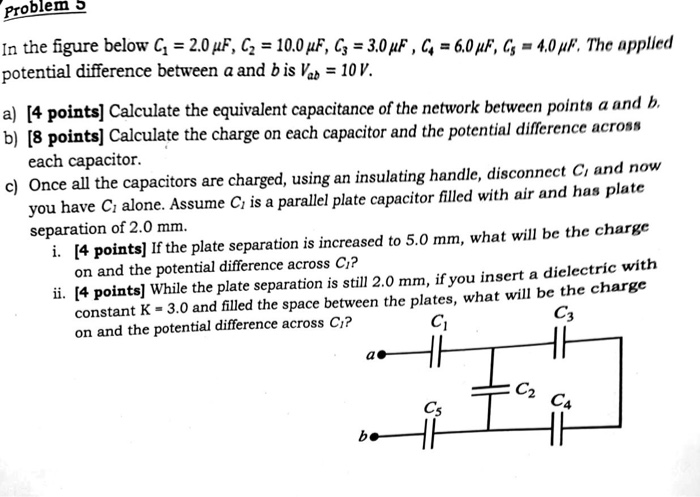Problem 5 In the figure below C1 2.0 μF, G 10.0pE, C3 3,0 μF , C.-6.0 μF.G-4.0 μ. The applied potential difference between a and b is Vab 10V. a) [4 points] Calculate the equivalent capacitance of the network between points a and b. b) [8 pointsl Calculate the charge on each capacitor and the potential difference across each capacitor. c) Once all the capacitors are charged, using an insulating handle, disconnect Ci and now you have C alone. Assume C is a parallel plate capacitor filled with air and has plate separation of 2.0 mm. i. [4 points] If the plate separation is increased to 5.0 mm, what will be the charge on and the potential difference across Ci? ii. [4 points] While the plate separation is still 2.0 mm, if you insert a dielectric with constant K -3.0 and filled the space between the plates, what wil be the charge on and the potential difference across Ci? с. Ci C4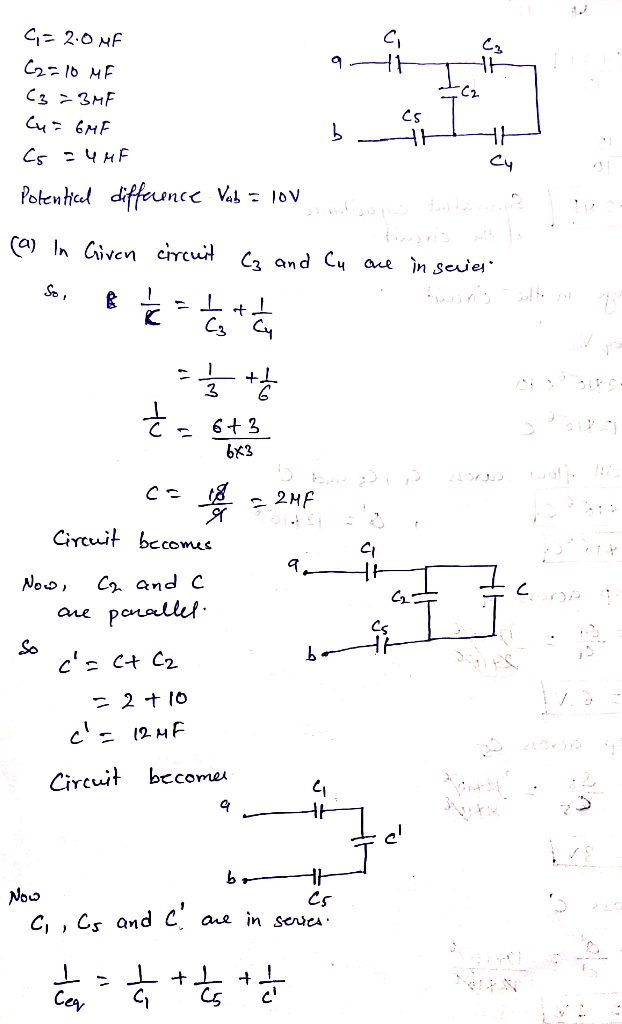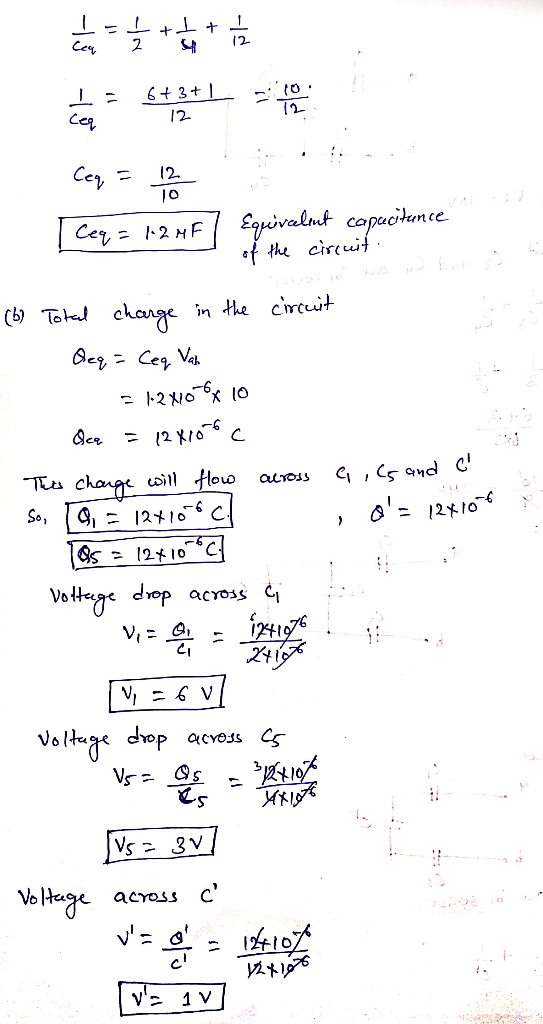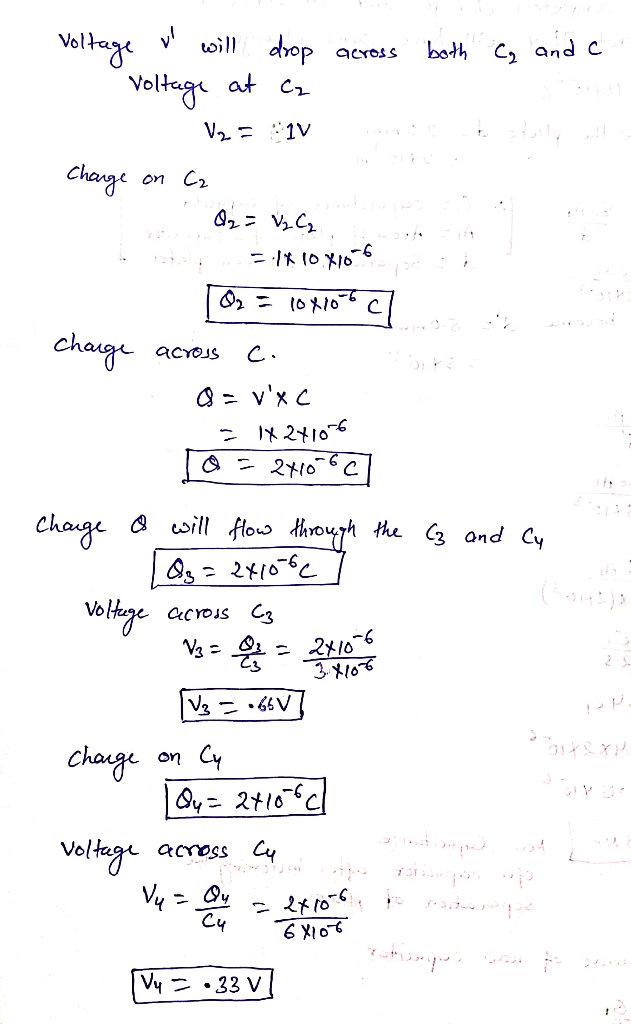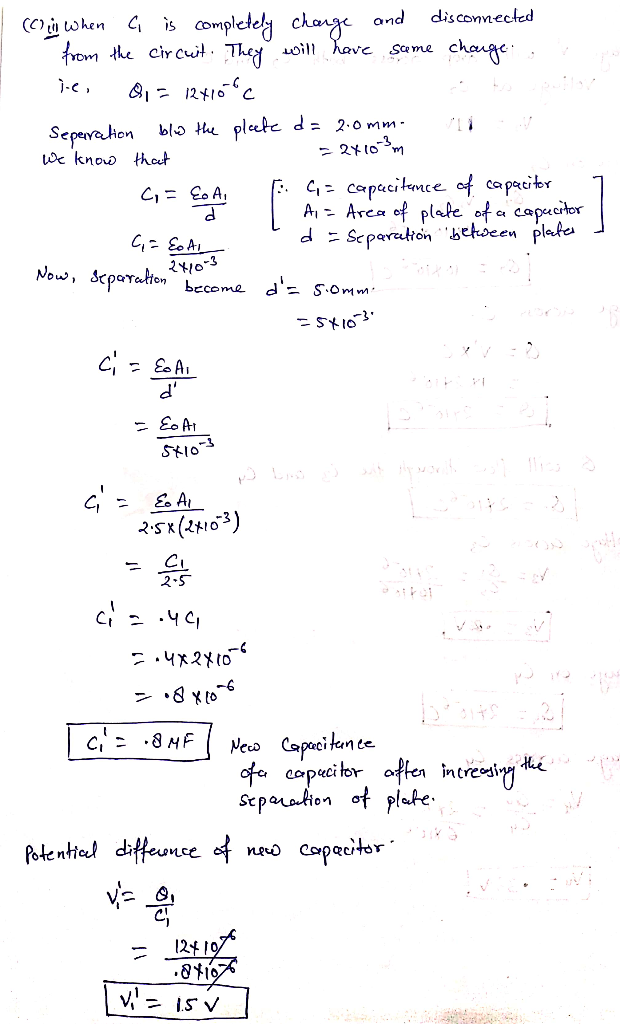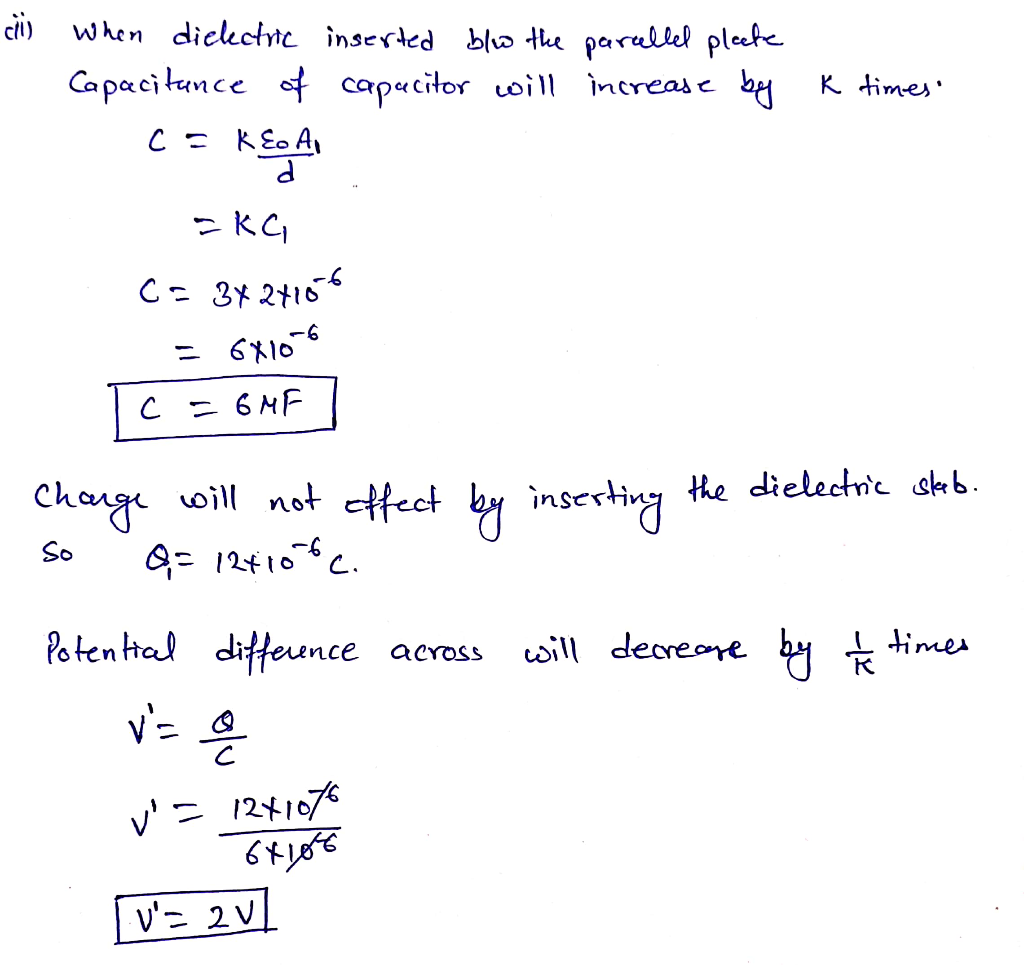​​​​​​

#### Earn Coins

Coins can be redeemed for fabulous gifts.

Similar Homework Help Questions
• ### Problem 5 In the figure below C1 2.0 μF, G 10.0pE, C3 3,0 μF , C.-6.0...Problem 5 In the figure below C1 2.0 μF, G 10.0pE, C3 3,0 μF , C.-6.0 μF.G-4.0 μ. The applied potential difference between a and b is Vab 10V. a) [4 points] Calculate the equivalent capacitance of the network between points a and b. b) [8 pointsl Calculate the charge on each capacitor and the potential difference across each capacitor. c) Once all the capacitors are charged, using an insulating handle, disconnect Ci and now you have C alone. Assume...

• ### In the figure (Figure 1), C1 = C5 = 8.3 μF and C2= C3 = C4 = 4.3 μF. The applied potential is Vab = 250 V.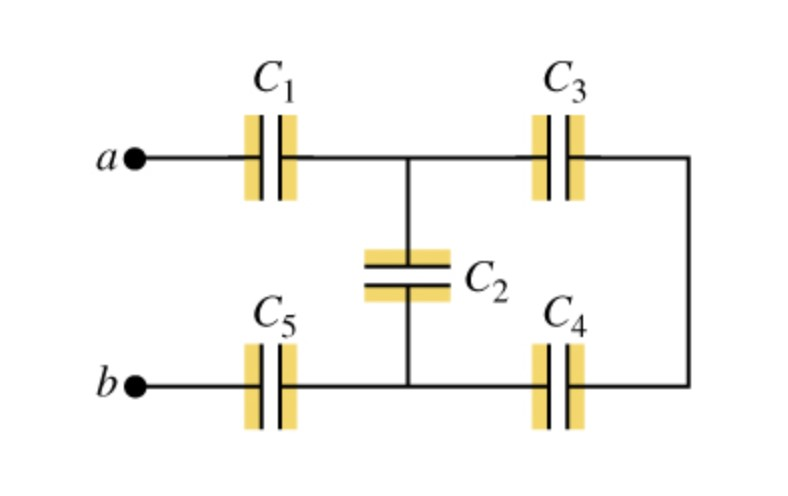In the figure (Figure 1), C1 = C5 = 8.3 μF and C2= C3 = C4 = 4.3 μF. The applied potential is Vab = 250 V.a) What is the equivalent capacitance of the network between points a and b?b) Calculate the charge on capacitor C1.c) Calculate the potential difference across capacitor C1.d) Calculate the charge on capacitor C2.e) Calculate the potential difference across capacitor C2.f) Calculate the charge on capacitor C3.g) Calculate the potential difference across capacitor C3.h) Calculate...

• ### Constanta In the figure (Figure 1), each capacitor has 5.00 μF and Vab = 33.0 Figure...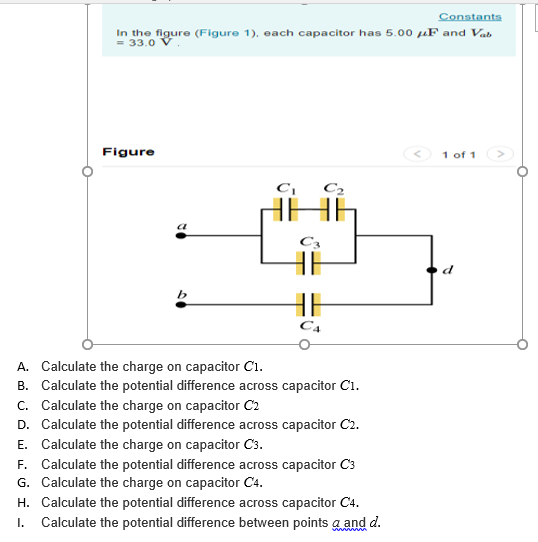Constanta In the figure (Figure 1), each capacitor has 5.00 μF and Vab = 33.0 Figure 1 of 1 CL A. B. C. D. E. F. G. H. I. Calculate the charge on capacitor Ci. Calculate the potential difference across capacitor Ci. Calculate the charge on capacitor C2 Calculate the potential difference across capacitor C2. Calculate the charge on capacitor C3. Calculate the potential difference across capacitor C3 Calculate the charge on capacitor C4. Calculate the potential difference across capacitor...

• ### C2 C3 2. In a circuit show on the left, C1 = 4.00 μF; C 4.00...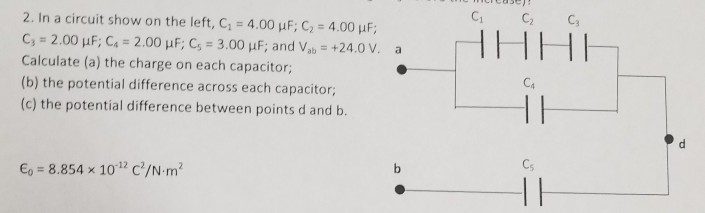C2 C3 2. In a circuit show on the left, C1 = 4.00 μF; C 4.00 c, = 2.00 μF; C4 = 2.00 μF; Cs = 3.00 μF; and Vab = +24.0 V. Calculate (a) the charge on each capacitor; (b) the potential difference across each capacitor (c) the potential difference between points d and b. a Ca Cs E.-8.854 × 10-12 C2/N·m2

• ### Constants PartA In the figure (Figure 1), G-G-8.5 μF and C2-C3 -G-4.3 R. The applied potential...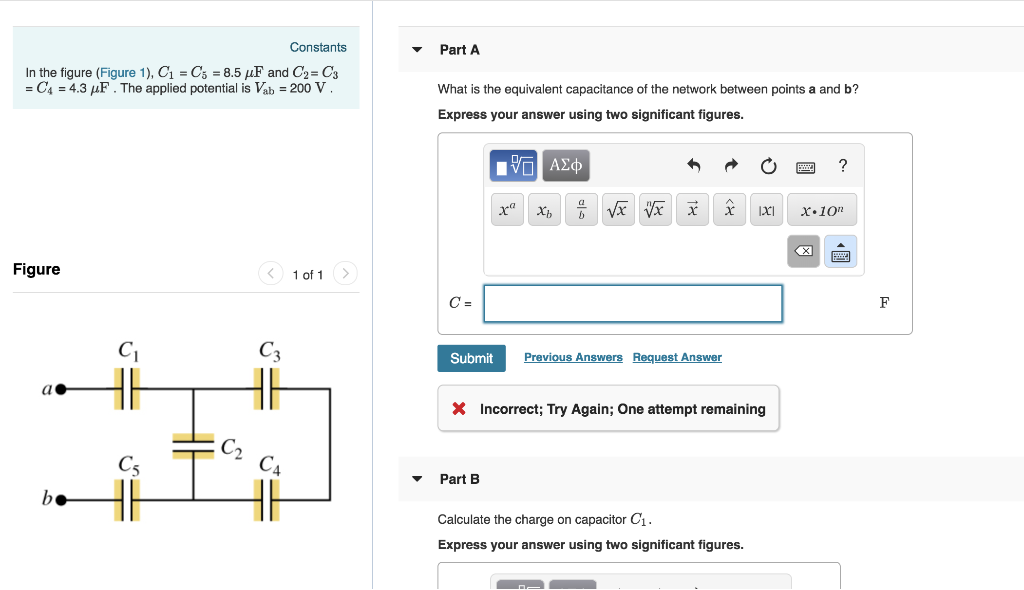Constants PartA In the figure (Figure 1), G-G-8.5 μF and C2-C3 -G-4.3 R. The applied potential is Kb-200 V What is the equivalent capacitance of the network between points a and b? Express your answer using two significant figures. Figure of 1 Ci C3 Submit Incorrect; Try Again; One attempt remaining C4 Part B Calculate the charge on capacitor C1. Express your answer using two significant figures. Part G Calculate the potential difference across capacitor C3 . Express your answer...

• ### As shown in the cireuit to the right, a potential difference of 625 V is applied...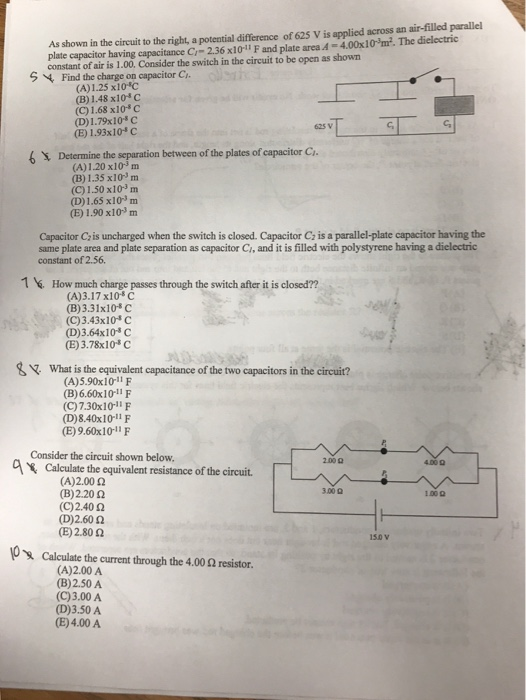As shown in the cireuit to the right, a potential difference of 625 V is applied across an air-filled parallel plate capacitor having capacitance Cr-2.36 x101l F and plate area 4-4.00x10m2. The dielectric constant of air is 1.00, Consider the switch in the circuit to be open as shown S 4 Find the charge on capacitor C (A)1.25 x10*C (B) 1.48 x10 c (C) 1.68x10 (D) 1.73x10-C (E) 1.93x10*c 625 v G, 6 Determine the between of the plates of...

• ### 1. The potential in a region between x-Oand x-600 mis V-e+bs, where α-198V and b -5.50...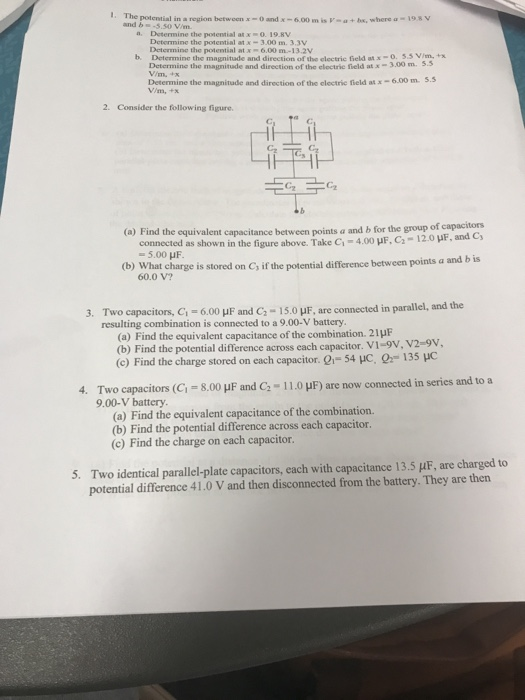1. The potential in a region between x-Oand x-600 mis V-e+bs, where α-198V and b -5.50 V/m a. Determine the potential atxo. 19.8V Determine the potential at x-3.00 m. 3.3V Determine the potential at x 6.00 m-13.2V Determine themagnitude and direction of the electric field Determine the magnitude and direction of the electric field at x-3.00 m5 Determine the magnitude and direction of the electric field at x-6.00 m. 5.5 Vim b. -0.53 2. Consider the following figure C2 (a)...

• ### v3. At what distance from a point charge of 4.0 nC does the electric field have...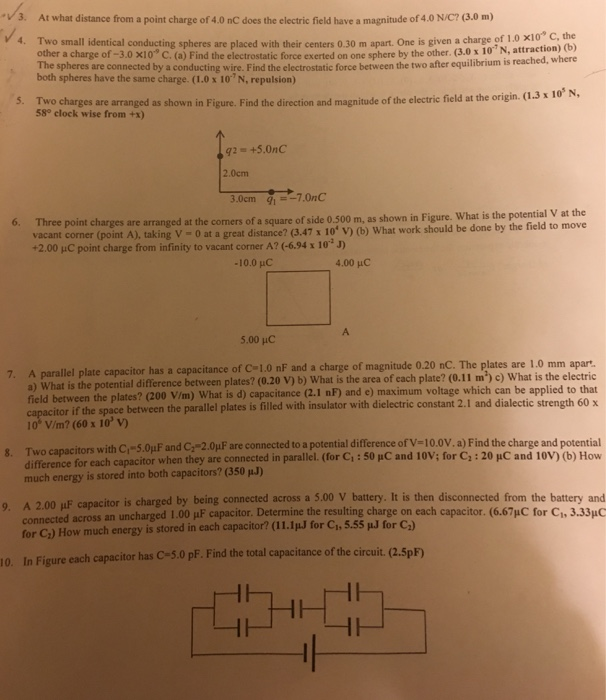v3. At what distance from a point charge of 4.0 nC does the electric field have a magnitude of 4.0 N/C? (3.0 m) 4. Two small identical conducting spheres are placed with their centers 0.30 m apart. One is given a charge of 1 0 x10* c other a charge of -3.0 x10 C. (a) Find the electrostatic force exerted on one sphere by the other. (3.0 x 10"N, attraction) the (b) e spheres are connected by a conducting wire....